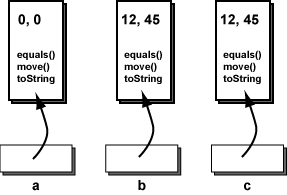What are the values of `x` and `y` in the third point?

x = 12 and y = 45.

# Picture of the ObjectsThe `Point`s were constructed with the statements

```    a = new Point();
b = new Point( 12, 45 );
c = new Point( b );
```

The constructor for point `a` automatically uses (0, 0).

The constructor for point `b` uses data from its two parameters.

The constructor for point `c` is given a reference to another point. The `x` and `y` values of the new point `c` will take their values from `b` as explained in the Point, class documentation.

After the three objects have been created (just before the program closes) the situation looks like the picture.

Each reference variable refers to an object. Each object contains its own data and its own methods.

### QUESTION 6:

Look at the picture. Is it clear what the phrase "the object `a`" means?